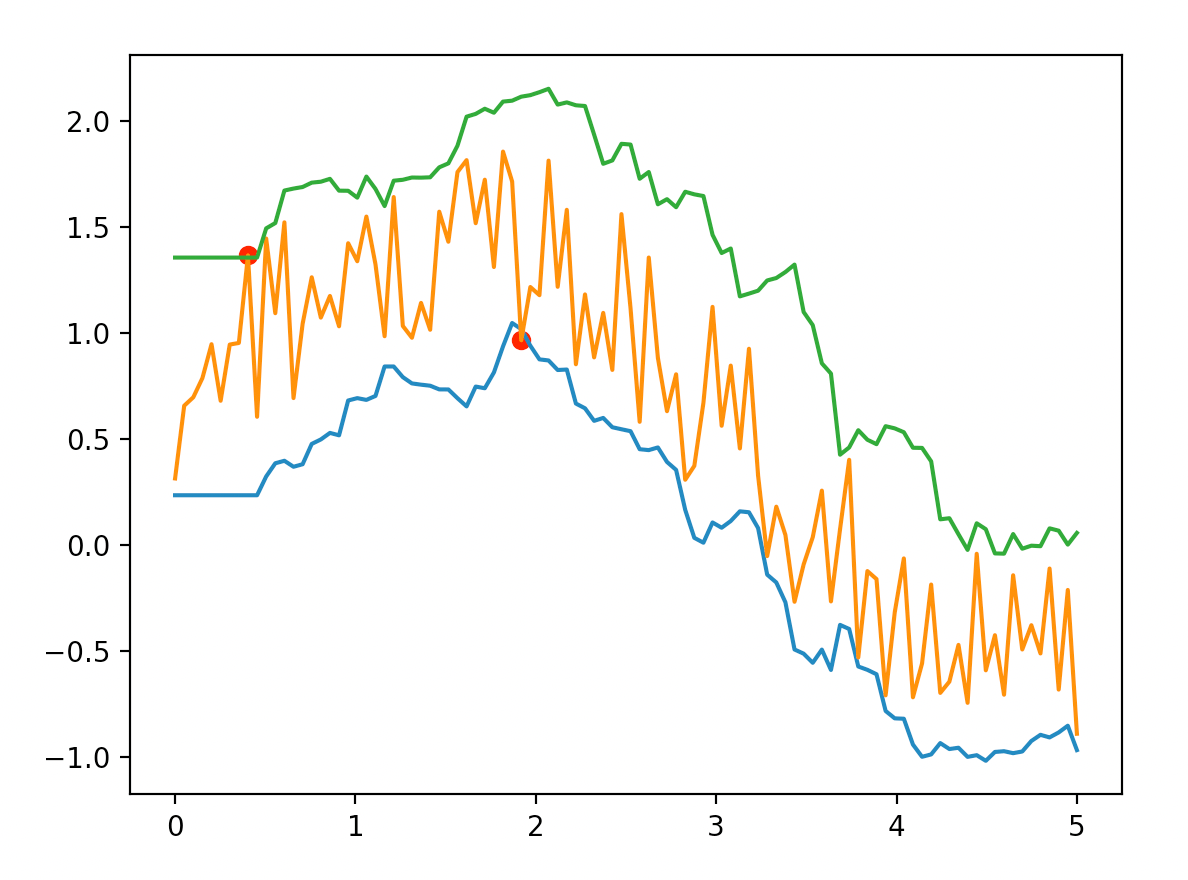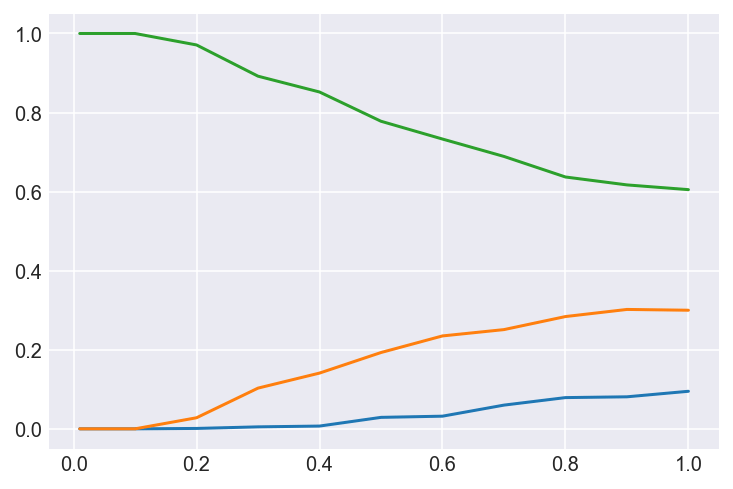## How to compute and plot Bollinger Bands® in Python

The aim is to produce a plot like this. The orange line is your data, the green line is the upper "bollinger" band, the blue line is the lower "bollinger" band. The red dots indicate where your data is either above or below the bands.Copy-paste this code:

``````import pandas as pd
import numpy as np
from matplotlib import pyplot as plt

N = 100
XMAX = 5
WINMA = 10
ALPHA = 2

def get_bollinger(data, winma=10, alpha=2):
ser = pd.Series(data)
ma = ser.rolling(winma).mean()
std = ser.rolling(winma).std()
lower = pd.Series(ma - alpha*std).fillna(method='bfill').values
upper = pd.Series(ma + alpha*std).fillna(method='bfill').values
return lower, upper

low = np.argwhere(data < lower)
high = np.argwhere(data > upper)
return low, high

if __name__=='__main__':

X = np.linspace(0.0, XMAX, num=N)
data = np.sin(X) + np.random.random(N)
lower, upper = get_bollinger(data, winma=WINMA, alpha=ALPHA)
low, high = get_alerts(data, lower, upper)
for i in low:
plt.plot(X[i], data[i], 'ro')
for i in high:
plt.plot(X[i], data[i], 'ro')
plt.plot(X, lower)
plt.plot(X, data)
plt.plot(X, upper)
plt.show()``````

## How to scrape images from the web

I'm interested in object detection and other computer vision tasks. For example, I'm working on a teddy-bear detector with my son.

So, how do you quickly download images for a certain category? You can use this approach that I learned from a course on Udemy.

``````# pip install icrawler

keywords = ['cat', 'dog']
for keyword in keywords:
storage={'root_dir': 'images/{}'.format(keyword)}

)
keyword=keyword, max_num=10, min_size=(200, 200))``````

In the above example, the crawler will find images in two categories -- cats and dogs, as if you search for 'cat' and 'dog' on Google images and downloaded what you found.

Let's walk through the parameters used in the code. First, there is the constructor, which is called with three arguments in the example. The most important parameter is storage, which specifies where the images will be stored. Second, we have the call to the crawl function. Here, the max_num parameter is used to specify that at most 10 images per category should be downloaded. The min_size argument specifies that the images must be at least 200 x 200 pixels.

## How to sample from softmax with temperature

Here is how to sample from a softmax probability vector at different temperatures.

```import numpy as np import matplotlib.pyplot as plt import matplotlib as mpl import seaborn as sns   mpl.rcParams['figure.dpi']= 144   trials = 1000 softmax = [0.1, 0.3, 0.6]   def sample(softmax, temperature): EPSILON = 10e-16 # to avoid taking the log of zero #print(preds) (np.array(softmax) + EPSILON).astype('float64') preds = np.log(softmax) / temperature #print(preds) exp_preds = np.exp(preds) #print(exp_preds) preds = exp_preds / np.sum(exp_preds) #print(preds) probas = np.random.multinomial(1, preds, 1) return probas   temperatures = [(t or 1) / 100 for t in range(0, 101, 10)] probas = [ np.asarray([sample(softmax, t) for _ in range(trials)]).sum(axis=0) / trials for t in temperatures ]   sns.set_style("darkgrid") plt.plot(temperatures, probas) plt.show()```

Notice how the probabilities change at different temperatures. The softmax probabilities are [0.1, 0.3, 0.6]. At the lowest temperatures of 0.01, the dominant index (value 0.6) has near 100% probability of being sampled. At higher temperatures, the selection probabilities move towards the softmax values, e.g. 60% probability for the third index.## How to do backpropagation in Numpy

I have adapted an example neural net written in Python to illustrate how the back-propagation algorithm works on a small toy example.

My modifications include printing, a learning rate and using the leaky ReLU activation function instead of sigmoid.

```import numpy as np   # seed random numbers to make calculation # deterministic (just a good practice) np.random.seed(1) # make printed output easier to read # fewer decimals and no scientific notation np.set_printoptions(precision=3, suppress=True)   # learning rate lr = 1e-2   # sigmoid function def sigmoid(x,deriv=False): if deriv: result = x*(1-x) else: result = 1/(1+np.exp(-x)) return result   # leaky ReLU function def prelu(x, deriv=False): c = np.zeros_like(x) slope = 1e-1 if deriv: c[x<=0] = slope c[x>0] = 1 else: c[x>0] = x[x>0] c[x<=0] = slope*x[x<=0] return c   # non-linearity (activation function) nonlin = prelu # instead of sigmoid   # initialize weights randomly with mean 0 W = 2*np.random.random((3,1)) - 1   # input dataset X = np.array([ [0,0,1], [0,1,1], [1,0,1], [1,1,1] ]) # output dataset y = np.array([[0,0,1,1]]).T   print('X:\n', X) print('Y:\n', y) print()   for iter in range(1000):   # forward propagation l0 = X l1 = nonlin(np.dot(l0,W))   # how much did we miss? l1_error = y - l1   # compute gradient (slope of activation function at the values in l1) l1_gradient = nonlin(l1, True) # set delta to product of error, gradient and learning rate l1_delta = l1_error * l1_gradient * lr   # update weights W += np.dot(l0.T,l1_delta)   if iter % 100 == 0: print('pred:', l1.squeeze(), 'mse:', (l1_error**2).mean())   print ("Output After Training:") print ('l1:', np.around(l1))```

I work in the ad tech industry, which means that I track people online for a living. Mainly, I do it because the industry has interesting computer science problems and because the job pays well.
I will not defend ad tech. Mainly because ad tech is not important enough to humanity to defend. However, I do believe that ad tech’s algorithms are important to humanity because they can be applied to important areas, such as your health, personal finance and education. However, I have a different point today.

Here is how it works. You get assigned a random number, e.g. 123, to represent you. Then, ad tech will attempt to link your number, 123, with the numbers of boxes that represent products or services that you might be interested in. For example, a box A could be people who need a vacation and box B could be people who could be tempted to buy a new BMW. Ideally, if you really need a vacation and someone really wants to sell you that vacation, then a connection between 123 and A should be made. From ad tech’s perspective, the number 123 is linked to the box A. The algorithm does not need to use labels like “Alice Anderson” or “Bob Biermann”, because the numbers 1 and 2 will get the job done just fine -- from a mathematical point of view.

At some point your true identity becomes interesting, long after ad tech has left the scene. At some point, somebody (e.g. a human being or a robot) might need to print your real name and street address on a card box box, put the product you ordered inside and ship it via DHL. Up until that exact point, your name, street address or any other personally identifiable information is utterly unimportant to anybody. Nobody cares and no advertisement algorithm needs to know. I think this is an important point.
Of course it is true that your real identity can be learned from seemingly anonymised data. It might even be fairly trivial to do so, using good old detective skills. Differential privacy has some fairly hard results in that area. However, the main point is that someone has to make a conscious decision to look into the data on a mission to find you and possibly design a new algorithm for that purpose.

Now I get to my main point. Yes, ad tech CAN know who you are with some detective work. However, ad tech does not NEED to know who you are in order to work. This is so important because it means that we can potentially harness the power of algorithms in areas of life that matter — without compromising the privacy of anybody. It is not going to be easy to obtain the granular and self-controlled privacy that is needed, but it is worthwhile. And that is why I joined ad tech in the first place, because the computer science problems are interesting and important — and well, interesting and important things tend to pay well.

## Neural networks on GPUs: cost of DIY vs. Amazon

I like to dabble with machine learning and specifically neural networks. However, I don't like to wait for exorbitant amounts of time. Since my laptop does not have a graphics card that is supported by the neural network frameworks I use, I have to wait for a long time while my models git fitted. This is a problem.

The solution to the problem is to get access to a computer with a supported Nvidia GPU. Two approaches are to either get my own rig or rent one from Amazon. Which is cheaper?

## Cost analysis

I will assume that I will train models on my machine (whether local or at Amazon) for two hours every day.

The Amazon p2 range of EC2 machines come with Nvidia K80 cards, which costs about 50.000 DKK. Already this analysis is going to be difficult; I will not buy a computer that costs 50.000 DKK just to train NN models. So, in this analysis I will be comparing apples to oranges, but that is how it is.

### Cost of Amazon

The p2.xlarge EC2 instance has a single K80 GPU, which is at least as good as any rig I would consider buying.

The on-demand prie is \$0.9/hour; the spot price about five times cheaper. Usage for two hours every day for a whole year costs 4.500 DKK for on-demand and 900 DKK for spot instances. However, the p2 instances is sometimes unavailable in the European spot markets.

### Cost of DIY

What is the best GPU to get for a DIY machine learning rig? In 2016, Quora answers suggested that the Nvidia cards Titan X and GTX980TI would be best. Let's go with that.

This is quite a bit more than 4.500 DKK and that is only for the graphics card. The finished rig would probably cost around 15.000 DKK (Titan) and 10.000 DKK (GTX).

The electricity also has to be factored in, plus that the cards are basically slower than the K80.

### Best choice for increased usage

With increased usage the DIY approach will become cheaper than Amazon, albeit still a slower option. With usage of 5 or 7 hours/day the DIY approaches break even after a year.

## How AI, robotics and advanced manufacturing could impact everybody’s life on Earth

What if everybody could live a wealthy, healthy, job-less and creative life in a post-scarcity Universe? Are we currently on a trajectory to this new reality and what are the obstacles we may face on the way? What are the important game-changing technologies?

TODO: create and agenda (very tentative):

1) contrast current life circumstances with a potential future
2) identify the key problems that we could solve with technology
3) review the players in society that will take part in this change
3) contrast views on the opportunities and threats of these technologies
4) ...

Our future life conditions here on Earth might soon be impacted by game-changing advancements in artifical intelligence, robotics, manufacturing and genetics; at least if you ask people like Elon Mush, Andrew Ng and Ray Kurzweil. What are the most important technologies and what is the impact they might have? What are the dangers? Opinions differ so the intention here is to review and contrast what leading fiction writers, scientists, visionaries and entrepreneurs think about the question: how will AI, robots, and advanced manufacturing impact everybody's life circumstances here on Earth?

## Fiction to be reviewed

Post-scarcity:
- The Culture series

AI:
- Asimov

The Human-Computer Cortex:
- That Swedish guy who wrote sci-fi computer implants in the 70's

AI:

## Videos to be reviewed

AI:

The Human-Computer Cortex:

## News articles to be reviewed

AI:
- https://aifuture2016.stanford.edu/
- http://fortune.com/2016/06/15/future-of-work-2/

3D printing:
- https://hbr.org/2013/03/3-d-printing-will-change-the-world

## When to be most careful about catching the flu?

Continuing on my blogification of Peter Norvigs excellent talk, the question is, when to watch out for the flu, e.g. if you live in Denmark?